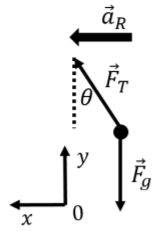$$\require{cancel}$$

6.7: Problems and Solutions

Exercise $$\PageIndex{1}$$

Consider a conical pendulum with a mass $$m$$, attached to a string of length $$L$$. The mass executes uniform circular motion in the horizontal plane, about a circle of radius $$R$$, as shown in Figure $$\PageIndex{1}$$. One can think of the horizontal circle and the point where the string is attached to as forming a cone. The circular motion is such that the (constant) angle between the string and the vertical is $$\theta$$.

1. Derive an expression for the tension in the string.
2. Derive an expression for the speed of the mass.
3. Derive an expression for the period of the motion.Figure $$\PageIndex{1}$$: The conical pendulum.

a. We start by identifying the forces that are acting on the mass. These are:

• $$\vec F_g$$, its weight, with a magnitude $$mg$$.
• $$\vec F_T$$, a force of tension exerted by the string.

The forces are illustrated in Figure $$\PageIndex{2}$$, along with our choice of coordinate system and the direction of the acceleration of the mass (towards the center of the circle).Figure $$\PageIndex{2}$$: Forces acting on the conical pendulum.

The $$y$$ component of Newton’s Second law gives the relation between the tension in the string, the weight, and the angle $$\theta$$ \begin{aligned} \sum F_y&=0 \\ F_T\cos\theta -F_g&=0 \\ F_T\cos\theta&=mg \\ \therefore F_T&=\frac{mg}{\cos\theta} \\\end{aligned}

b. In order for the mass to move in a circle, the net force must be directed towards the center of the circle at all times. The $$x$$ component of Newton’s Second Law, combined with our expression for the magnitude of the tension, $$F_T$$, allows us to determine the speed of the mass: \begin{aligned} \sum F_x&=ma_r \\ F_T\sin\theta&=m\frac{v^2}{R}\\ \left(\frac{mg}{\cos\theta}\right)\sin\theta &=m\frac{v^2}{R}\\ g\tan\theta&=\frac{v^2}{R}\\ \therefore v &= \sqrt{gR\tan\theta}\end{aligned}

c. Now that we know the speed, we can easily find the period, $$T$$, of the motion: \begin{aligned} T&=\frac{2\pi R}{v} \\ &=\frac{2\pi R}{\sqrt{gR\tan\theta }}=2\pi\sqrt{\frac{R}{g\tan\theta}}\end{aligned}

Exercise $$\PageIndex{2}$$

Barb and Kenny are going to the amusement park. Barb insists on riding the giant roller coaster, but Kenny is scared that they will fall out of the roller coaster at the top of the loop. Barb reassures Kenny by asking the roller coaster technician for more information. The technician says that they will be traveling at $$15\text{m/s}$$ when upside down, and that the roller coaster loop has a radius of $$22\text{m}$$. Kenny is still skeptical. Is he correct in being skeptical?Figure $$\PageIndex{3}$$: The roller coaster
Writing Newton’s Second Law in the vertical direction, for the case where only the weight acts on Barb or Kenny (mass $$m$$), when they are going at speed $$v$$ \begin{aligned} mg &= ma_R = m\frac{v^2}{R}\\ \therefore v &= \sqrt{gR} = \sqrt{(9.8\text{m/s}^{2})(22\text{m})}=14.68\text{m/s}\end{aligned} This corresponds to the minimum speed that they must have at the top of the loop to make it around. If they go faster, the normal force from their seat (downwards, since they are upside-down), would result in a larger net force towards the center of the circle. This situation corresponds to the normal force from their seat just barely reaching 0 at the top of the loop. Since the roller coaster is quoted as having a speed of $$15\text{m/s}$$ at the top of the loop, they will just barely make it. However, this is way too close to the minimal speed to not fall out of the roller coaster, so Kenny is correct in being skeptical! The engineers designing the roller coaster should include a much bigger safety margin!